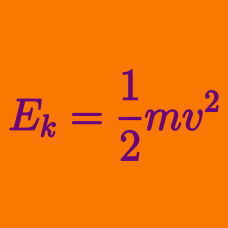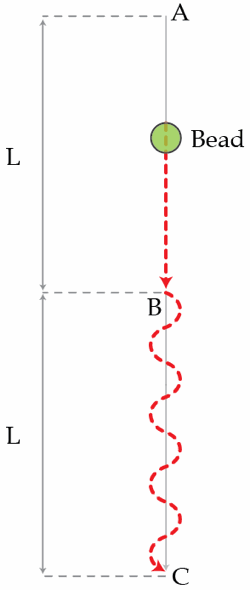Classical Mechanics

# Kinetic Energy: Level 2-4 Challenges

Hydrovolts has invented a water powered cell phone charger - the HydroBee. Put the charger in a flow of water, like a stream or faucet, and a little turbine spins, generates electricity, and charges up your phone. Hence if you are out camping you can now charge a phone without carrying along a bunch of batteries. If the HydroBee has a circular opening aperture of radius $5~\mbox{cm}$ and is placed in a stream with flow rate $1~\mbox{m/s}$, how long in hours will it take to completely charge an empty cell phone battery rated at $5~\mbox{W-h}$ (Watt-hours)?

Details and assumptions

• The density of water is $\rho=1~\mbox{g/cm}^3$.
• The energy conversion efficiency of the kinetic energy of the water to electric energy is 50%.
• The water flows through the entire opening aperture.

I spin a frisbee on my finger about the center of the frisbee. I then hit the side of the frisbee to make it spin twice as fast as originally. By what factor has the kinetic energy of the frisbee increased?

The caber toss is a traditional Scottish sport that involves hurling a caber, which is essentially a large piece of a tree: a caber is a $6~\mbox{m}$ long, $80~\mbox{kg}$ tree trunk. Large strong people hurl these and the goal is to get the caber to land as far away as possible and rotate in the air, so what was the highest part of the trunk initially is actually the part that hits the gound first. See this clip to understand how the caber rotates.

It's HARD to do this. To see how hard, consider a perfectly vertical caber with one end on the ground. You then launch the caber vertically with some speed $v_0$ and give it a rotation. What is the minimum kinetic energy in Joules you need to give the caber so that when it lands the caber is perfectly vertical again, but the OTHER end of the caber hits the ground?

Details and assumptions

• The acceleration of gravity is $-9.8~\mbox{m/s}^2$.
• The caber can be modeled as a uniform rod.

Three identical point charges, each with mass $m=1~\text{g}$, are connected by strings of the same length $l$ so that they form an equilateral triangle ABC. Initially, the charges are at rest and the electrostatic potential energy of the system is $U_{0}=1~\text{J}$. Suddenly, the string connecting the charges at A and B snaps. What will be the maximum speed in meters per second of the charge located at C.

A bead slides down on a frictionless wire, starting from rest at point A. The section from A to B is straight while the section from B to C is wavy (with wavelength $\lambda\ll L$). The total length of the stretched wire is $3L$. If $L=50~\text{cm}$, determine the time in seconds it takes for the bead to reach point C. Assume that the bead slides smoothly at all times.Details and assumptions

$g=9.8~\text{m}/\text{s}^2$

×

Problem Loading...

Note Loading...

Set Loading...